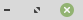https://dev.webpages.dk/PHP Update a Database
PHP Update a Database:To update some row with existing values, in a table, you will use the UPDATE statement.

The examples to the right will do exactly that. Something like this:

UPDATE tablename SET field=value

You should make use of the WHERE clause, if you omit this the update may affect all the field values in the table.

### Update a Database

How do you Update content to a Database using PHP?

Next step in this short serie is to make an UPDATE in our existing data. If you copy the code below into a PHP document you should be able to UPDATE your table rows. Put the code in a file named 'update.php' and call it in your browser.

The last part of the code is what we actually see first.
It will populate a SELECT with our contacts. We also get a submitbutton with the caption "Okay, select".

When we click that button we go to the function doread(). Here we read what AutoID number was selected, it is the number of the contact we have selected, so when we have that we can read all info from the database in the row containing the values for our selected contact.
We get the values presented in text-fields, so we can change the text as we like. Then when we click this button, we read (in function doedit()) the inputs from the textfields on the HTML form, we put the content into some variables, in this example five variables.

We now construct the SQL, where we update the database fields, with the inputs from the form contained in the variables.
We make sure to put things in the right place, and we do that by stating that we want to update the fields in the row WHERE AutoID is the \$IDnummer. That was the value that we read from the 'Hidden' form field.

Next I will look at how to make order in the search results from querying you database table.

### HTML

``````<HTML><BODY>
<FORM METHOD='POST' ACTION=''>
<TABLE>
<TR>
<TD>Our contacts: </TD>
``````
``````
<?php
\$con = mysqli_connect('127.0.0.1', 'root', 'Your_Password', 'dbname');

if (mysqli_connect_errno()) {
echo 'Error connecting to DB';
}

\$SQL_String = mysqli_query(\$con, 'SELECT * FROM contacts ORDER BY AutoID ASC');

while(\$row = mysqli_fetch_array(\$SQL_String))  {
echo '<OPTION VALUE=' . \$row['AutoID'] . '>' . \$row['Firstname'] . ' ' . \$row['Lastname'];
}
mysqli_close (\$con);
?>
``````
``````
</SELECT>
</TD>
</TR>
<TR>
<TD></TD>

</TR>
</TABLE>
</FORM>
</BODY></HTML>``````

### PHP

``````<?php
if (isset(\$_POST['updaterow']))  {
doedit();
}

}

function doedit() {

\$IDnummer = \$_POST['idnummer'];
\$Firstname = \$_POST['firstname'];
\$Lastname = \$_POST['lastname'];

\$con = mysqli_connect('127.0.0.1', 'root', 'Your_Password', 'dbname');

if (mysqli_connect_errno()) {
echo "Error connecting to the DB";
}

\$SQL_String = "UPDATE contacts SET Firstname='\$Firstname',
Lastname='\$Lastname',
WHERE AutoID='" . \$IDnummer . "'";

mysqli_query(\$con, \$SQL_String);
mysqli_close (\$con);

}

\$IDnummer = \$_POST['contact'];

echo "<HTML><BODY><FORM METHOD='POST' ACTION=''>
<INPUT TYPE='hidden' NAME='idnummer' VALUE='" . \$IDnummer . "'>
<TABLE>";

\$con = mysqli_connect('127.0.0.1', 'root', 'Your_Password', 'dbname');
if (mysqli_connect_errno()) {
echo 'Error connecting to DB';
}
\$SQL_String = mysqli_query(\$con, 'SELECT * FROM contacts WHERE AutoID=' . \$IDnummer . '');
\$row = mysqli_fetch_array(\$SQL_String);

echo "<TR>
<TD>Firstname: </TD>
<TD><INPUT TYPE='text' NAME='firstname' VALUE=" . \$row['Firstname'] . "></TD>
</TR>";

echo "<TR>
<TD>Lastname: </TD>
<TD><INPUT TYPE='text' NAME='lastname' VALUE=" . \$row['Lastname'] . "></TD>
</TR>";

echo "<TR>
</TR>";

echo "<TR>